# Automatic Modulation Classification Using a Support Vector Machine-Based Pattern Recognition Algorithm

Automatic Modulation Classification Using a Support Vector Machine-Based Pattern Recognition Algorithm
Department of ECE, Vels Institute of Science, Technology & Advanced Studies, Chennai 600117, India

Corresponding Author Email:
meena.se@velsuniv.ac.in
Page:
999-1007
|
DOI:
https://doi.org/10.18280/isi.270617
22 October 2022
|
Accepted:
3 December 2022
|
Published:
31 December 2022
| Citation

OPEN ACCESS

Abstract:

Modulation format recognition is an essential part of intelligent receivers of wireless communication systems, especially for adaptive radio systems (ARS). This paper presents a detailed investigation of automatic modulation classification (AMC) using pattern recognition classifiers (PRC) under fading and AWGN conditions. A variety of classifiers with different kernel functions and Support Vector Machine (SVM) classifiers have been developed for the classification of higher-order digital modulation signals. In addition, an extensive investigation of the extraction of various higher-order statistical features from each of the modulated classes and the choice of appropriate features for training classifiers are presented. In addition, the performance of the SVM classifier is evaluated under a variety of training rates and suboptimal channel conditions. Further, the performance of SVM classifiers is compared to that of existing techniques to demonstrate the effectiveness of the SVM classifiers for modulation categorization.

Keywords:

modulation recognition, support vectors, kernel function, linear and non-linear kernels, cumulants

1. Introduction

Early research on AMC focuses only on basic continuous modulations, thereafter, with the evaluation of wireless communication systems, attention is shifting to digital modulations. Broadly, the AMC techniques are categorised into decision-theoretic (DT) or maximum likelihood (ML) approaches and feature-based (FB) or pattern recognition (PR) approaches. The likelihood functions of an unknown signal are compared to those of the available set using the Generalized and Averaged Likelihood Ratio Tests (ALRT & GLRT) [4, 5]. In DT methods [6-10], the choice is made by calculating the likelihood ratio of the unknown signal against a threshold that is constructed with different algorithms. The threshold is formulated by extracting known signal features. The DT approaches are ineffective and computationally more complex when signal parameters are unknown, but these ML classifiers' performances are optimal when the signal parameters are known prior. However, prior knowledge of signal waveform characteristics is impractical in real-time applications. On the other side, the FB approaches [11-16] classify the signals based on signal statistical features. The classification process has two phases: the feature extraction phase and the classification phase. Even though DT approaches are optimal, their computational complexity is higher than that of the FB approaches. The FB approaches may be optimal if the proper feature set is chosen.

From the detailed literature, it is also found that some of the other approaches have also been developed for AMC. They are statistical approaches [17-22], where different statistical features of the signal, such as correlations, moments, and cumulants in the complex envelope of the signal, are extracted and then a multilevel classification algorithm is applied for classifying the signals. The accuracy of the Back Propagation Neural Network (BPNN) is higher than that of the Kolmogorov Smirnov (KS) and higher-order statistics (HoS) approaches . SVM classifiers are used in several applications, such as speech recognition, text classification, data classification, etc. [24, 25].

It is evident from the critical review of the literature that good research has already been done by several researchers to improve the performance of AMC methods under different noisy conditions. The techniques that are discussed in existing literature have the following limitations: The major limitations of decision-theoretic approaches are the formulation of the right hypothesis and the consideration of the optimum threshold value. Prior knowledge about signal and channel characteristics is necessary to get optimum performance, which is impractical [26, 27]. Also, these algorithms are computationally complex, and they are sensitive to phase, frequency offsets, and synchronisation errors. whereas the selection of the right feature set is the major limitation of statistical approaches and wavelet transform approaches. These feature sets have been significantly affecting the performance of the classifier. Further, most of the existing FB methods are developed at a constant SNR level. Very often, time-varying noise channels and multipath fading channels degrade the performance of AMC. No attempt has been made so far to achieve high classification accuracy along with a low-complexity solution by considering all classes of digital modulation schemes. In this paper, an efficient and robust SVM classifier for AMC in next-generation adaptive radio systems is implemented. Noise-insensitive features are identified using Principle Component Analysis (PCA) to ensure the classifier is robust with SNR variations as well as with multipath fading effects. The performance of SVM classifiers with linear, polynomial, and gaussian kernels for AMC is analysed with all possible combinations of training rates and SNR conditions. In this paper, a variety of SVM classifiers are developed and analysed for AMC.

The rest of the paper is organised as follows: the framework of the proposed approach is discussed in Section 2. Section 3 describes the SVM algorithm for AMC. The simulation results of an SVM based AMC algorithm in non-ideal channel conditions are discussed in Section 4. Finally, Section 5 depicts the important conjectures of the paper.

2. System Model

Figure 1 represents the outline of the proposed method. It consists of training and testing phases. To analyze the performance, a set of modulation classes (1,000 samples of each modulation class) is generated under different noisy conditions. Based on the training rate parameter, some portion of the data set is separated for training, and the rest is used for testing.

## 1.png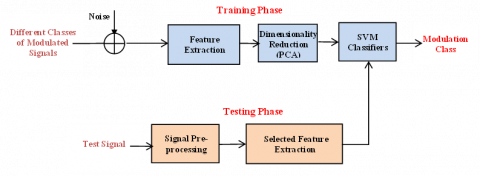Figure 1. Proposed system model

2.1 Signal model

The input signal for the CR receiver r(n) is given by

$r(n)=x(n)+g(n)$        (1)

here, x(n) is the signal which is transmitted and g(n) is fading in the channel.

For binary data input, the r(n) is :

$r(n)=A e^{i\left(2 \pi n T f_o+\theta_n\right)} \sum_{k=-\infty}^{\infty} x(k) y((n-k+\epsilon) T)$          (2)

here, A is the amplitude, x(k) is input binary stream, y(.) is channel effects, T is symbol time, θn is phase jitter, and $\epsilon$  is time shifts due to channel characteristics.

2.2 Feature extraction

To train the classifier, various statistical features are extracted for each set of modulated classes under non-ideal channel conditions. Moments are monotonically increasing functions for the "M" value in M-ary PSK and QAM modulated signals. Higher order modulated signals have higher values of moments, so these moments are suitable for the classification of digital signals.

For a received signal r(n) the moments are extracted as :

$M_{p q}=E\left[r(n)^{p-q} r^*(n)^q\right]$      (3)

here, p, q are the integers, r(n) is the received signal, and r*(n) is the complex conjugate of the received signal.

The cumulants of a signal are a set of statistical quantities that derived from moments. The HoC are derived from moments and further, these are used for deriving the ratios . There are 39 features were identified from the literature which are popularly used, and they are shown in Table 1.

2.3 Feature selection

From the observations of the derived statistical features of all modulation classes in the feature extraction stage, it is found that some of the moments and higher-order cumulant ratios are undesirable to distinguish between modulation classes. For some of the modulation classes, moments and higher-order ratios have the same range of values. Due to all these moments and cumulant ratios, the classification time is increased without any progress in the accuracy. To reduce the complexity of the classifier, Principle Component Analysis (PCA) is carried out on all 39 features. Through the PCA, finally, a set of 11 best features is selected for the best classification accuracy. The 11 features are cumulants of the second, fourth, sixth, and eight orders, and they are further used for training the SVM classifier.

2.4 Training the classifier & testing

In the training phase, the proposed classifiers are trained with the selected feature set of all the reference modulated signals. In this phase, six different types of SVM classifiers are trained with the identified features discussed in 2.3. The detailed training process of SVM is presented in Section 3.

Finally, in the testing phase, from an unknown received signal, selected features are extracted for AMC after signal preprocessing. The extracted features are further passed through the trained classifier for modulation reorganization. Based on the model built by the classifier in the training phase, it classifies the unknown signal into a particular class using the extracted feature set. Here, the classification accuracy (A) is given by:

$A=\frac{1}{n} \sum_{i=0}^n P\left(C_i \mid C_i\right)$           (4)

here, P(Ci|Ci) is probability of classification of a class Ci into class Ci and n is the number of classes.

Table 1. Statistical Features for AMC

 Moments Cumulants Higher order ratios $M_{20}=E\left[r(n)^2\right]$ $M_{21}=E[r(n) r *(n)]$ $M_{22}=E\left[r^*(n)^2\right]$ $M_{40}=E\left[r(n)^4\right]$ $M_{41}=E\left[r(n)^3 r^*(n)^1\right]$ $M_{42}=E\left[r(n)^2 r^*(n)^2\right]$ $M_{43}=E\left[r(n)^1 r^*(n)^3\right]$ $M_{60}=E\left[r(n)^6\right]$ $M_{61}=E\left[r(n)^5 r^*(n)^1\right]$ $M_{62}=E\left[r(n)^4 r^*(n)^2\right]$ $M_{63}=E\left[r(n)^3 r^*(n)^3\right]$ $M_{80}=E\left[r(n)^8\right]$ $M_{84}=E\left[r(n)^4 r^*(n)^4\right]$ $C_{20}=E\left[r^2(n)\right]$ $C_{21}=E\left[|r(n)|^2\right]$ $C_{40}=M_{40}-3 M_{20}{ }^2$ $C_{41}=M_{40}-3 M_{20} M_{21}$ $C_{42}=M_{42}-2 M_{21}-\left|M_{21}\right|^2$ $C_{60}=M_{60}+30 M_{20}{ }^3-15 M_{20} M_{40}$ $C_{61}=M_{61}+30 M_{20}^2 M_{21}-10 M_{20} M_{41}$ $C_{62}=M_{62}-6 M_{20} M_{42}+24 M_{21}^2 M_{22}-8 M_{21} M_{41}+6 M_{20}{ }^2 M_{22}-M_{22} M_{40}$ $C_{63}=M_{63}+18 M_{20} M_{21} M_{22}+12 M_{21}{ }^3-9 M_{21} M_{42}-3 M_{20} M_{43}-3 M_{22} M_{41}$ $C_{80}=M_{80}-630 M_{20}{ }^4+420 M_{40} M_{20}{ }^2-28 M_{60} M_{20}-35 M_{40}{ }^2$ $C_{84}=M_{84}-16 C_{63} C_{21}+\mid C_{40 \mid}{ }^2-18 C_{42}{ }^2-72 C_{42} C_{21}^2-24 C_{21}{ }^4$ $r_1=\left|C_{40}\right| /\left|C_{42}\right|$ $r_2=\left|C_{41}\right| /\left|C_{42}\right|$ $r_3=\left|C_{42}\right| /\left|C_{21}\right|^2$ $r_4=\left|C_{60}\right| /\left|C_{21}\right|^3$ $r_5=\left|C_{63}\right| /\left|C_{21}\right|^3$ $r_6=\left|C_{60}\right|^2 /\left|C_{42}\right|^3$ $r_7=\left|C_{63}\right|^2 /\left|C_{42}\right|^3$ $r_8=\left|C_{80}\right| /\left|C_{21}\right|^2$ $r_9=\left|C_{84}\right| /\left|C_{21}\right|^2$ $r_{10}=\left|C_{80}\right| /\left|C_{21}\right|^3$ $r_{11}=\left|C_{84}\right| /\left|C_{21}\right|^3$ $r_{12}=\left|C_{80}\right| /\left|C_{42}\right|^2$ $r_{13}=\left|C_{84}\right| /\left|C_{42}\right|^2$ $r_{14}=\left|C_{80}\right| /\left|C_{42}\right|^3$ $r_{15}=\left|C_{84}\right| /\left|C_{42}\right|^3$
3. SVM Classifier for AMC

The SVM classifier has the ability to classify high-dimensional and noisy data. It is a supervised algorithm that classifies the data using a subset of training samples. It takes a known data set to build a model, which is then used to classify the unknown data. The SVM classifier creates a feature space with the help of the training data. Thereafter, it tries to identify a hyperplane that divides the plane into two parts, where each half contains only one class.

## 2.png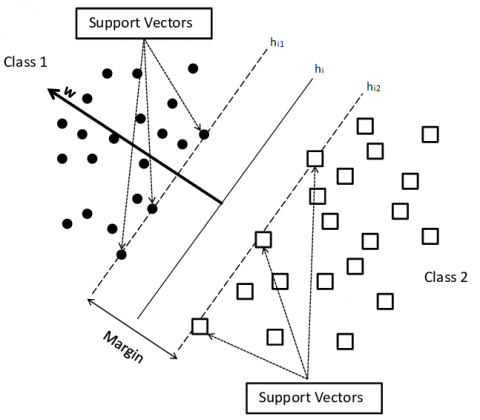Figure 2. Margin and decision borders of SVM classifier

Figure 2 represents the typical classification with SVM classifier. It consists of two classes with two different symbols circles and square boxes. The classifier finds a “maximum margin hyper-plane” as the best decision margins to separates the data. For every plane hi there exist a pair of supporting hyper-planes hi1 and hi2 which is parallel to hi. The distance between hi1 and hi2 is called as margin. To construct the hyper plane SVM follows two principles, and they are selecting the best Hyper-plane for classification, and it should be the maximum distance between two supporting planes.

SVM classifiers classified as linear and nonlinear SVMs based on kernel functions used for classification.

3.1 Linear SVM classifier

To distinguish between the classes, a linear kernel is considered in linear SVM and it is given as:

$F(a, w)=a^T w$          (5)

where, w is the weight vector and a is the input feature vector.

The hyper plane with linear kernel and a constant w0 is given by:

$h(a)=a^T w+w_0$      (6)

The binary classification is illustrated in Figure 3.

The decision for the Figure 3 is defined as:

$M= \begin{cases}M(P), & \text { if } h(a) \geq 0 \\ M(Q), & \text { if } h(a)<0\end{cases}$       (7)

where, P and Q are two classes, the class is recognized as member (M) of P if aTw+w0≥0, else it is recognized as Q.

Let $w$ be a vector in $\Re^d$ and $\Delta\left(a, w_0\right)=$ $\left\{S \in \Re^d \mid a^T w+w_0=0\right\}$ is a hyperplane then the distance between vector $w$ and the hyperplane $\Delta\left(a, w_0\right)$ is $\operatorname{dist}\left(w_i, \Delta\left(a, w_0\right)\right)=\frac{\left|a^T w+w_0\right|}{\|a\|}$.

To get optimal accuracy the optimization of weight is:

maximize $S\left(w, w_0\right)=\frac{1}{\|w\|^2}$      (8)

To maximize S the condition to be fallowed is:

$x_j(h(a)) \geq 1, \quad j=1,2, \ldots . N$         (9)

here, for M(Q) xj is -1 and +1 for M(P). And xj indicates the modulation class for the feature vector j.

## 3.png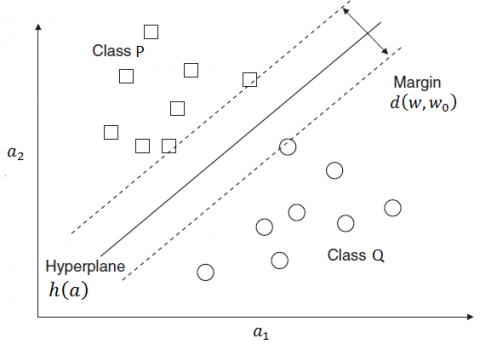Figure 3. Classification using Linear SVM

3.2 Nonlinear SVM classifiers

In this work, non-linear kernel’s such as polynomial and Gaussian are used for classification of two modulation classes, and they are given as:

$K(P, Q)=\left(\gamma \cdot P^T Q+r\right)^d, \gamma>0$      (10)

The degree of polynomial is given by d and it represents the polynomial kernel.

$K(P, Q)=\exp \left(\|P-Q\|^2 / 2 \sigma^2\right)$        (11)

here, $\sigma$ varies with the number of features and it represents the Gaussian kernel.

Based on the degree SVM classifiers with polynomial kernel are classified as Cubic SVM and Quadratic SVM.

The kernel function for Cubic SVM and Quadratic SVM are defined as:

$K(P, Q)=\left(\gamma \cdot P^T Q+r\right)^3, \gamma>0$             (12)

$K(P, Q)=\left(\gamma \cdot P^T Q+r\right)^4, \gamma>0$            (13)

## 4.png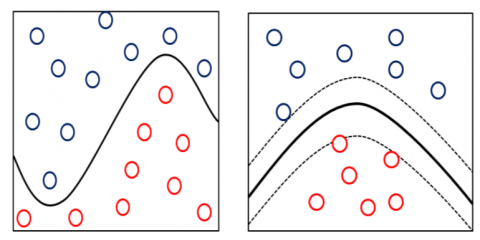Figure 4. SVM binary classification with cubic and quadratic kernels

Figure 4 represents the binary classification using cubic and quadratic kernels. Based on the $\sigma$ value used in Gaussian kernel the SVM classifiers are classified as Fine, Medium and Coarse Gaussian SVMs. The $\sigma$ values for Fine gaussian is $\frac{\sqrt{P}}{4}$ similarly for medium gaussian kernel $\sigma$ is $\sqrt{P}$ and for coarse gaussian is $4 \sqrt{P}$. The kernel representation fine, medium and coarse Gaussian SVM are shown in Figure 5.

To verify the performance of SVM classifier, the modulation classification is carried with the linear, cubic, quadratic, fine, medium and coarse Gaussian kernels.

## 5.png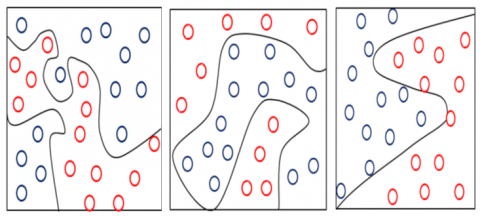Figure 5. SVM binary classification with fine, medium and coarse Gaussian kernels

4. Simulation Results and Discussions

To analyze the performance of proposed SVM classifiers, a set of six different modulated signals, each with 1000 copies, is considered under varying noise conditions such as fading and AWGN with an SNR of 0 to 20 dB. The modulation classes considered for experimental simulations are M-ary QAM (with M = 4, 16, and 64) and M-ary PSK (M=2, 4, and 8). To classify the modulation classes, a set of 11 features that are discussed in feature selection are extracted for each modulated signal. Thereafter, the feature set of size 6000*12 (11 features and one label) was divided into a training set and a testing set.

Initially, the performance analysis was carried out with 90% of the feature set as a training set, and the remaining 10% as testing data. Further, the analysis is extended to a training set of 80%, 70%, 60%, and 50%. To verify the superiority of the proposed classifier, the performance is compared with the standard benchmark functions such as ML, AMPT, GLRT, HoC, KS, and BPNN.

Table 2 and Table 3 represent the confusion matrix for different SVM classifiers using multi-order cumulants at different SNRs. Diagonal elements in the confusion matrix denote the true classification rates, and off-diagonal elements represent misclassification rates

The average performance accuracy of Linear SVM (LSVM) and Quadratic SVM (QSVM) classifiers for different modulation is shown in Figure 6. The average modulation classification accuracy of Linear SVM and Quadratic SVM at SNR 0 dB are 82.8% and 83.1% respectively.

The performance accuracy of proposed Cubic SVM and Fine Gaussian SVM (FGSVM) classifiers for different modulation classes and at different SNR values is shown in Figure 7. The average modulation classification accuracy of Cubic SVM and FGSVM classifiers are at SNR 0 dB is 81.7% and 82.5% respectively.

The average performance accuracy of proposed Medium Gaussian SVM (MGSVM) and Coarse Gaussian SVM (CGSVM) classifiers for different modulation classes and at different SNR values is shown in Figure 8. The average modulation classification accuracy of MGSVM and CGSVM classifiers is at SNR 0 dB is 82.8% and 80.6% respectively.

Figure 9 depicts the performance comparison of proposed SVM classifiers. QSVM and CSVM classifiers attains better classification performance i.e., polynomial kernel provides better classification than that of linear and gaussian kernels.

Table 2. Confusion matrix for proposed SVM classifiers with 90% training (0dB and 5 dB and 10dB)

 Classifier True Class SNR 0 dB 5 dB 10 dB Linear SVM BPSK 100 0 0 0 0 0 100 0 0 0 0 0 100 0 0 0 0 0 QPSK 0 90 0 0 3 7 0 100 0 0 0 0 0 100 0 0 0 0 8PSK 0 0 83 0 10 7 0 0 93 0 7 0 0 0 100 0 0 0 4QAM 0 0 0 100 0 0 0 0 0 100 0 0 0 0 0 100 0 0 16QAM 0 0 13 0 51 37 0 0 3 0 90 7 0 0 0 0 97 3 64QAM 0 0 0 0 27 73 0 3 0 0 4 93 0 0 0 0 0 100 Quadratic SVM BPSK 100 0 0 0 0 0 100 0 0 0 0 0 100 0 0 0 0 0 QPSK 4 90 0 0 3 3 0 100 0 0 0 0 0 100 0 0 0 0 8PSK 0 0 80 0 7 13 0 0 100 0 0 0 0 0 100 0 0 0 4QAM 0 0 0 100 0 0 0 0 0 100 0 0 0 0 0 100 0 0 16QAM 0 0 17 0 46 37 0 0 3 0 87 10 0 0 0 0 97 3 64QAM 0 0 0 0 17 83 0 0 0 0 3 97 0 0 0 0 0 100 Cubic SVM BPSK 100 0 0 0 0 0 100 0 0 0 0 0 100 0 0 0 0 0 QPSK 3 90 0 0 0 7 0 100 0 0 0 0 0 100 0 0 0 0 8PSK 0 0 87 3 0 10 0 0 100 0 0 0 0 0 100 0 0 0 4QAM 0 0 0 100 0 0 0 0 0 100 0 0 0 0 0 100 0 0 16QAM 0 0 30 0 20 50 0 0 3 0 83 14 0 0 0 0 97 3 64QAM 0 0 3 0 4 93 0 0 0 0 3 97 0 0 0 0 0 100 Fine Gaussian SVM BPSK 100 0 0 0 0 0 100 0 0 0 0 0 100 0 0 0 0 0 QPSK 7 87 0 0 0 6 0 100 0 0 0 0 0 100 0 0 0 0 8PSK 3 0 77 0 7 13 3 0 90 0 0 7 3 0 97 0 0 0 4QAM 0 0 0 100 0 0 0 0 0 100 0 0 0 3 0 97 0 0 16QAM 0 3 13 0 51 33 0 0 3 0 87 10 0 0 3 0 90 7 64QAM 0 3 0 0 17 80 0 3 0 0 10 87 0 0 0 0 0 100 Medium Gaussian SVM BPSK 100 0 0 0 0 0 100 0 0 0 0 0 100 0 0 0 0 0 QPSK 3 90 0 0 0 7 0 100 0 0 0 0 0 100 0 0 0 0 8PSK 0 0 77 0 10 13 0 0 93 0 7 0 0 0 100 0 0 0 4QAM 0 0 0 100 0 0 0 0 0 100 0 0 0 0 0 100 0 0 16QAM 0 3 10 0 50 37 0 0 3 0 87 10 0 0 3 0 87 10 64QAM 0 3 0 0 13 80 0 3 0 0 10 87 0 0 0 0 0 100 Gaussian SVM BPSK 97 3 0 0 0 0 100 0 0 0 0 0 100 0 0 0 0 0 QPSK 3 87 0 0 3 7 0 100 0 0 0 0 0 100 0 0 0 0 8PSK 0 0 80 0 17 3 0 0 90 0 10 0 0 0 100 0 0 0 4QAM 0 0 0 100 0 0 0 0 0 100 0 0 0 0 0 100 0 0 16QAM 0 3 10 0 53 34 0 0 3 0 83 14 0 0 3 0 83 14 64QAM 0 10 0 0 23 67 0 3 0 0 10 87 0 0 0 0 0 100

Table 3. Confusion matrix for proposed SVM classifiers with 90% training (15 dB and 20 dB)

 Classifier True Class SNR 15 dB 20 dB Linear SVM BPSK 100 0 0 0 0 0 100 0 0 0 0 0 QPSK 0 100 0 0 0 0 0 100 0 0 0 0 8PSK 0 0 97 0 3 0 0 0 100 0 0 0 4QAM 0 0 0 100 0 0 0 0 0 100 0 0 16QAM 0 0 0 0 100 0 0 0 0 0 100 0 64QAM 0 0 0 0 0 100 0 0 0 0 0 100 Quadratic SVM BPSK 100 0 0 0 0 0 100 0 0 0 0 0 QPSK 0 100 0 0 0 0 0 100 0 0 0 0 8PSK 0 0 100 0 0 0 0 0 100 0 0 0 4QAM 0 0 0 100 0 0 0 0 0 100 0 0 16QAM 0 0 0 0 100 0 0 0 0 0 100 0 64QAM 0 0 0 0 0 100 0 0 0 0 0 100 Cubic SVM BPSK 100 0 0 0 0 0 100 0 0 0 0 0 QPSK 0 100 0 0 0 0 0 100 0 0 0 0 8PSK 0 0 97 0 3 0 0 0 100 0 0 0 4QAM 0 0 0 100 0 0 0 0 0 100 0 0 16QAM 0 0 0 0 100 0 0 0 0 0 100 0 64QAM 0 0 0 0 0 100 0 0 0 0 0 100 Fine Gaussian SVM BPSK 100 0 0 0 0 0 100 0 0 0 0 0 QPSK 0 100 0 0 0 0 0 100 0 0 0 0 8PSK 0 0 100 0 0 0 0 0 100 0 0 0 4QAM 0 0 0 100 0 0 0 0 0 100 0 0 16QAM 0 0 3 0 94 3 0 0 0 0 100 0 64QAM 0 0 0 0 0 100 0 0 0 0 0 100 Medium Gaussian SVM BPSK 100 0 0 0 0 0 100 0 0 0 0 0 QPSK 0 100 0 0 0 0 0 100 0 0 0 0 8PSK 0 0 97 0 3 0 0 0 100 0 0 0 4QAM 0 0 0 100 0 0 0 0 0 100 0 0 16QAM 0 0 0 0 97 3 0 0 0 0 100 0 64QAM 0 0 0 0 0 100 0 0 0 0 0 100 Gaussian SVM BPSK 100 0 0 0 0 0 100 0 0 0 0 0 QPSK 0 97 0 0 0 3 0 100 0 0 0 0 8PSK 0 0 97 0 3 0 0 0 100 0 0 0 4QAM 0 0 0 100 0 0 0 0 0 100 0 0 16QAM 0 0 0 0 93 7 0 0 0 0 100 0 64QAM 0 0 0 0 0 100 0 0 0 0 0 100

## 6a.png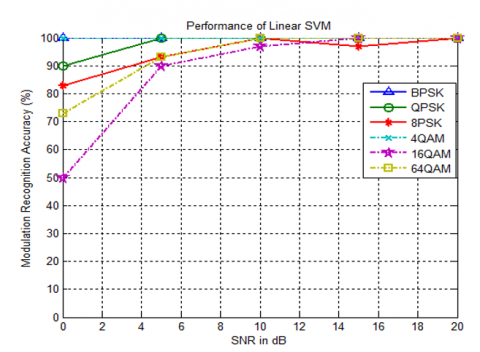(a)

## 6b.png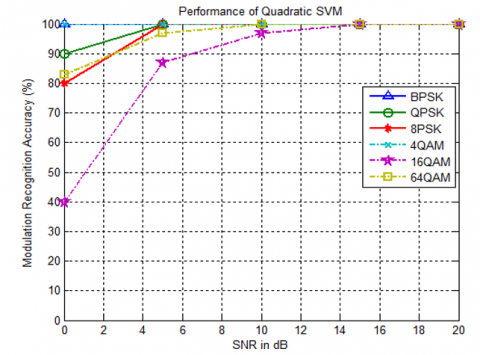(b)

Figure 6. Classification Accuracy of (a) LSVM and (b) QSVM Classifiers

## 7a.png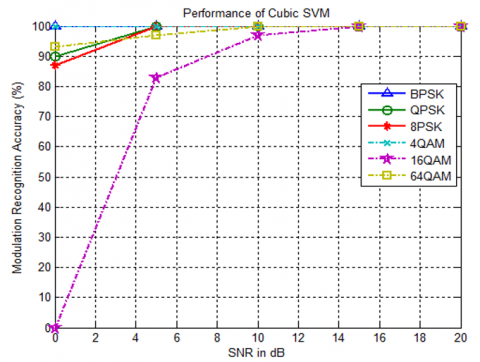(a)

## 7b.png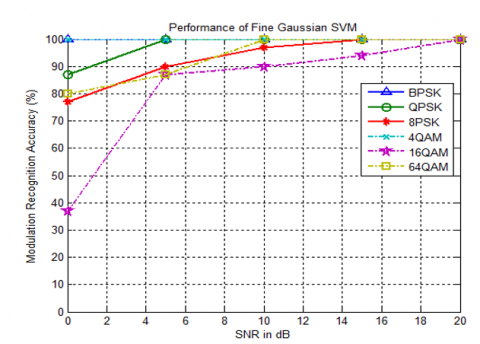(b)

Figure 7. Classification Accuracy of (a) Cubic and (b) FGSVM Classifiers

## 8a.png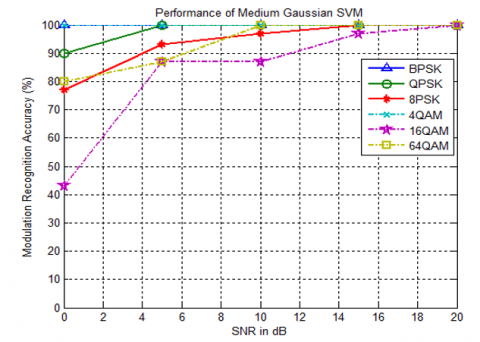(a)

## 8b.png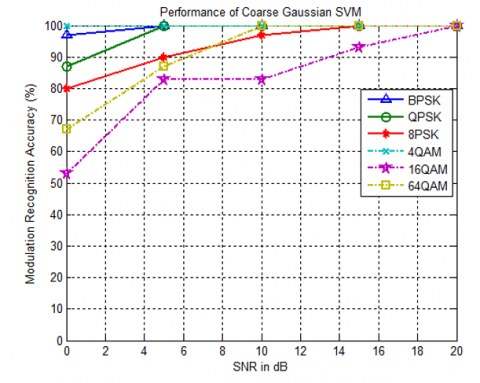(b)

Figure 8. Classification Accuracy of MGSVM and CGSVM Classifiers

## 9.pngFigure 9. Performance Comparison of all SVM Classifiers

## 10.pngFigure 10. Performance Comparison of SVM with Existing Classifiers

## 11.png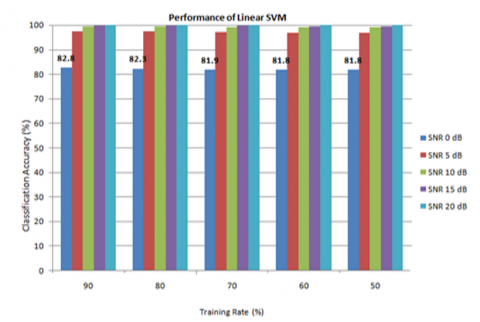Figure 11. Performance of LSVM

## 12.png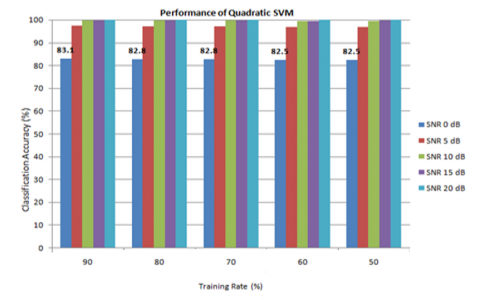Figure 12. Performance of QSVM

## 13.png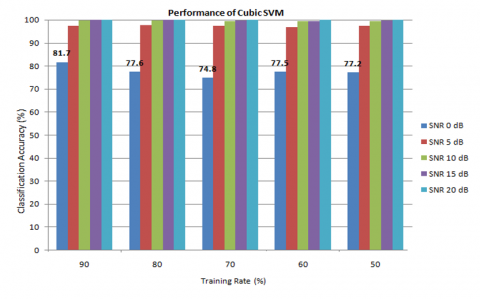Figure 13. Performance of CSVM

## 14.png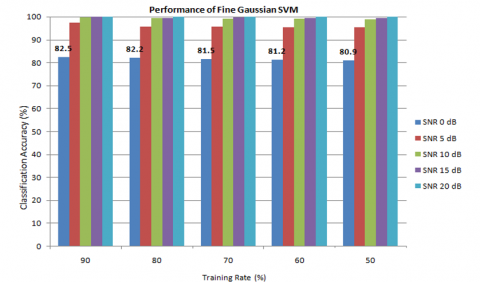Figure 14. Performance of FGSVM

## 15.png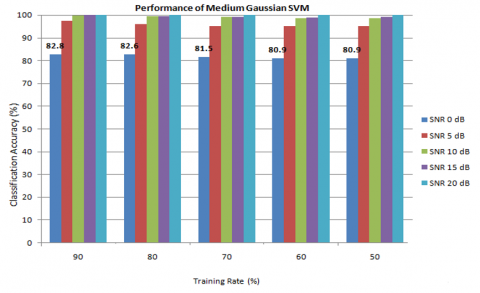Figure 15. Performance of MGSVM

## 16.png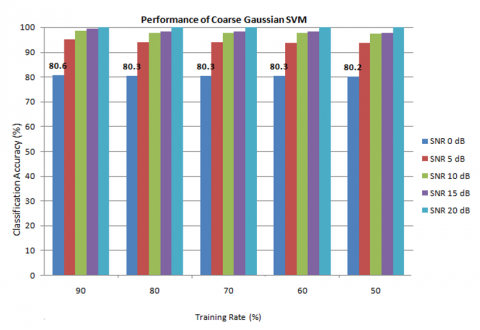Figure 16. Performance of CGSVM

Figure 10 depicts the performance comparison of proposed classifiers with that of the current techniques in the literature. From the comparison it is cleared that even at lower SNR values the SVM classifiers gives more recognition accuracy.

The performance of the proposed SVM classifiers with different training rates are shown in Figure 11 to Figure 16. For each type of SVM, the performance is plotted training rate versus performance accuracy for different SNR values. Figure 11 shows that the performance of linear SVM is consistent for less training rates and for SNR 0 dB.

The performance of Quadratic SVM is as shown in Figure 12. It is evident that even with 50 percent training the classification accuracy is higher.

From Figure 13 to Figure 16 represent the performance analysis of Cubic SVM, FGSVM, MGSVM, and CGSVM. From the analysis, it is evident that the performance of proposed SVM classifiers is consistent for all training rates and for all SNR values. CSVM and QSVM achieved higher accuracy i.e. polynomial kernel provides best classification with SVM classifier. The performance of proposed classifiers is better than the superior approaches in the existing methods such as HoC, ML, and BPNN.

5. Conclusions

In this work, a wide variety of SVM classifiers with different characteristics are developed for the classification of MQAM and MPSK signals. The extraction of statistical features for each modulation class and the selection of appropriate futures for training are presented. The performance of proposed SVM classifiers is analyzed under non-ideal channel conditions with various values of SNR and training rates. From the results, it is evident that the proposed SVM classifiers attain higher recognition accuracy even with less training and lower SNRs than existing approaches such as HoC, ML, and BPNN. From the performance analysis, it is evident that SVM with a polynomial kernel has superior performance to that of other kernels.

Further, this work can be extended by integrating SVM classifiers with optimization algorithms to get optimal accuracy. By establishing a complete communication link with Universal Software Radio Peripherals (USRPs), realistic signals are traced, and the proposed classifiers are applied for the analysis of the performance of proposed algorithms in real time applications.

References

 Rao, N.V., Krishna, B.T. (2022). Performance analysis of automatic modulation recognition using convolutional neural network. In Evolution in Signal Processing and Telecommunication Networks, pp. 443-452. https://doi.org/10.1007/978-981-16-8554-5_42

 Zhang, Z., Hua, Z., Liu, Y. (2017). Modulation classification in multipath fading channels using sixth-order cumulants and stacked convolutional auto-encoders. IET Communications, 11(6): 910-915. https://doi.org/10.1049/iet-com.2016.0533

 Subbarao, M.V., Samundiswary, P. (2018). Automatic modulation recognition in cognitive radio receivers using multi-order cumulants and decision trees. Int. J. Rec. Technol. Eng.(IJRTE), 7: 61-69.

 Wei, W., Mendel, J.M. (2000). Maximum-likelihood classification for digital amplitude-phase modulations. IEEE transactions on Communications, 48(2): 189-193. https://doi.org/10.1109/26.823550

 Panagiotou, P., Anastasopoulos, A., Polydoros, A. (2000). Likelihood ratio tests for modulation classification. In MILCOM 2000 Proceedings. 21st Century Military Communications. Architectures and Technologies for Information Superiority (Cat. No. 00CH37155), 2, pp. 670-674. https://doi.org/10.1109/MILCOM.2000.904013

 Dobre, O.A., Hameed, F. (2006). Likelihood-based algorithms for linear digital modulation classification in fading channels. In 2006 Canadian conference on electrical and computer engineering, pp. 1347-1350. IEEE. https://doi.org/10.1109/CCECE.2006.277525

 Wong, M.D., Nandi, A.K. (2006). Blind phase-amplitude modulation classification with unknown phase offset. In 18th International Conference on Pattern Recognition (ICPR'06), pp. 177-180. https://doi.org/10.1109/ICPR.2006.333

 Shimbo, D., Oka, I., Ata, S. (2007). A modulation classification using joint moments with linear transform. In 2007 IEEE Radio and Wireless Symposium, pp. 567-570. https://doi.org/10.1109/RWS.2007.351894

 Li, C., Xiao, J., Xu, Q. (2011). A novel modulation classification for PSK and QAM signals in wireless communication. In IET International Conference on Communication Technology and Application (ICCTA 2011), pp. 89-92. https://doi.org/10.1049/cp.2011.0636

 Abu-Romoh, M., Aboutaleb, A., Rezki, Z. (2018). Automatic modulation classification using moments and likelihood maximization. IEEE Communications Letters, 22(5): 938-941. https://doi.org/10.1109/LCOMM.2018.2806489

 Hu, S., Pei, Y., Liang, P.P., Liang, Y. C. (2019). Deep neural network for robust modulation classification under uncertain noise conditions. IEEE Transactions on Vehicular Technology, 69(1): 564-577. https://doi.org/10.1109/TVT.2019.2951594

 Wu, H.C., Saquib, M., Yun, Z. (2008). Novel automatic modulation classification using cumulant features for communications via multipath channels. IEEE Transactions on Wireless Communications, 7(8): 3098-3105. https://doi.org/10.1109/TWC.2008.070015

 Subbarao, M.V., Samundiswary, P. (2019). K-nearest neighbors based automatic modulation classifier for next generation adaptive radio systems. Int J. Security Appl., 13: 41-50.

 Subbarao, M.V., Samundiswary, P. (2018). Spectrum sensing in cognitive radio networks using time–frequency analysis and modulation recognition. Microelectronics, Electromagnetics and Telecommunications. Springer, Singapore, 827-837. https://doi.org/10.1007/978-981-10-7329-8_85

 Subbarao, M.V., Samundiswary, P. (2021). Automatic modulation classification using cumulants and ensemble classifiers. In Advances in VLSI, Signal Processing, Power Electronics, IoT, Communication and Embedded Systems, pp. 109-120. https://doi.org/10.1007/978-981-16-0443-0_9

 Wu, Z., Zhou, S., Yin, Z., Ma, B., Yang, Z. (2017). Robust automatic modulation classification under varying noise conditions. IEEE Access, 5: 19733-19741. https://doi.org/10.1109/ACCESS.2017.2746140

 Liu, D., Wang, P., Wang, T., Abdelzaher, T. (2021). Self-contrastive learning based semi-supervised radio modulation classification. In MILCOM 2021-2021 IEEE Military Communications Conference (MILCOM), pp. 777-782. https://doi.org/10.1109/MILCOM52596.2021.9652914

 Arjun, K.R., Surekha, T.P. (2021). Over-the-air modulation classification using deep learning in fading channels for cognitive radio. Indian Journal of Science and Technology, 14(46): 3360-3369. https://doi.org/10.17485/IJST/v14i46.2073

 Deshmukh, A., Narasimhadhan, A.V. (2022). Modulation and signal class labelling using active learning and classification using machine learning. arXiv preprint arXiv:2202.12930. https://doi.org/10.1109/CONECCT55679.2022.9865826

 Liu, P., Shui, P.L. (2014). A new cumulant estimator in multipath fading channels for digital modulation classification. IET Communications, 8(16): 2814-2824. https://doi.org/10.1049/iet-com.2014.0175

 Chang, D.C., Shih, P.K. (2015). Cumulants‐based modulation classification technique in multipath fading channels. Iet Communications, 9(6): 828-835. https://doi.org/10.1049/iet-com.2014.0773

 Alvarez, J.L.B., Montero, F.E.H. (2017). Classification of MPSK signals through eighth-order statistical signal processing. IEEE Latin America Transactions, 15(9): 1601-1607. https://doi.org/10.1109/TLA.2017.8015041

 Popoola, J.J., van Olst, R. (2011). Automatic classification of combined analog and digital modulation schemes using feedforward neural network. In IEEE Africon'11, pp. 1-6. https://doi.org/10.1109/AFRCON.2011.6072008

 Subbarao, M.V., Padavala, A.K., Harika, K.D. (2022). Performance Analysis of Speech Command Recognition Using Support Vector Machine Classifiers. In: Gu, J., Dey, R., Adhikary, N. (eds) Communication and Control for Robotic Systems. Smart Innovation, Systems and Technologies, vol 229. Springer, Singapore. https://doi.org/10.1007/978-981-16-1777-5_19

 Khare, V., Kumari, S. (2022). Performance comparison of three classifiers for fetal health classification based on cardiotocographic data. Acadlore Trans. Mach. Learn., 1(1): 52-60. https://doi.org/10.56578/ataiml010107

 Kumar, I., Mishra, M.K., Mishra, R.K. (2021). Performance analysis of NOMA downlink for next- generation 5G network with statistical channel state information. Ingénierie des Systèmes d’Information, 26(4): 417-423. https://doi.org/10.18280/isi.260410

 El Mettiti, A., Oumsis, M. (2022). A survey on 6G networks: Vision, requirements, architecture, technologies and challenges. Ingénierie des Systèmes d’Information, 27(1): 1-10. https://doi.org/10.18280/isi.270101